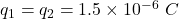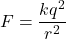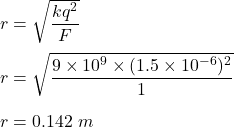## What is the distance between two spheres each with a charge of 1.5 x 10 -6C when the force between them is I N

Question

What is the distance between two spheres each with a charge of 1.5 x 10 -6C when the force between them is I N

in progress 0
8 months 2021-07-13T19:28:58+00:00 1 Answers 4 views 0

The distance between spheres is 0.142 meters.

Explanation:

We have,

Magnitude of charges isForce between charges, F = 1 N

It is required to find the distance between spheres having charges. The electrical force between charges is given by :r is distanceSo, the distance between spheres is 0.142 meters.